﻿ MotionDesigner Reference & User Interface > BLEND-POINT (NODE) EDITOR > Blend-Point Editor: Y-axis Values

# Blend-Point Editor: Y–axis Values

### Blend-Point Editor:  Y-axis Values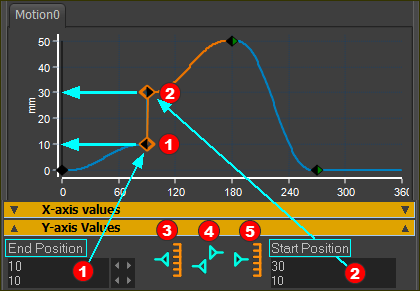Y-axis Values

There are Four Motion-Derivatives.

Note: Only the Flexible Polynomial can directly edit all of the motion-derivatives. There are limits with the other motion-laws.

 Position(P), Velocity(V), Acceleration(A), and Jerk(J).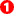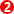Two Motion-Values (for each Motion-Derivative) :End (motion-value) of Previous Segment (Read-Only or Read-Write) E.g. In the image above, the motion-value at the: End Position of the Previous-Segment = 10mmStart (motion-value) of the Selected-Segment (Read-Only or Read-Write) E.g. In the image above, the motion-value at the: Start Position of the Selected-Segment = 30mm These data-boxes have two possible states: Read-Only or Read-Write The Motion-Law, Motion-Derivative and the Control-Buttons determine the state of the two data-boxes.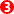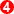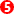Three Control Buttons (for each Motion-Derivative) :

See more at Control Buttons: States and PatternsEnd Control Button - to control if you can specify the motion-value at the End of the Previous-Segment. This button has three(3) possible states - Do Edit, Do Not Edit, and Can Not EditMatch Control Button - to control flow of motion-values from the Previous-Segment to the Selected-Segment . This button has two(2) possible states -   Do Force Start of the Selected-Segment = End of the Previous-Segment Do NOT Force Start of the Selected-Segment = End of the Previous-SegmentStart Control Button - to control if you can specify the motion-value at the Start-of the Selected-Segment. It has three(3) possible states - Do Edit, Do Not Edit, and Can Not Edit

### Example: Blend-Point Editor: Three(3) Control-Buttons with EACH Motion-Derivative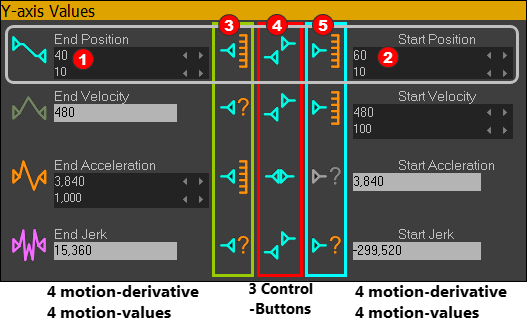In this example, the states of the 3 Control-Buttons for Position are:EDIT the motion-value ...at END Position of Previous-Segment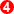DO NOT force the start Position to equal the end Position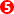EDIT the motion-value ...at START Position  of the Selected-Segment